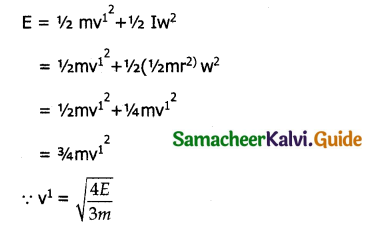Tamilnadu State Board New Syllabus Samacheer Kalvi 11th Physics Guide Pdf Chapter 5 Motion of System of Particles and Rigid Bodies Text Book Back Questions and Answers, Notes.

## Tamilnadu Samacheer Kalvi 11th Physics Solutions Chapter 5 Motion of System of Particles and Rigid Bodies

### 11th Physics Guide Motion of System of Particles and Rigid Bodies Book Back Questions and AnswersPart – I:
I. Multiple choice questions:

Question 1.
The center of mass of a system of particles does not depend upon, _______. [AIPMT 1997, AIEEE 2004]
a) position of particles
b) relative distance between particles
c) masses of particles
d) force acting on particle
d) force acting on particle

Question 2.
A couple produces, _______. [AIPMT 1997]
a) pure rotation
b) pure translation
c) rotation and translation
d) no motion
a) pure rotation

Question 3.
A particle is moving with a constant velocity along a line parallel to positive x-axis. The magnitude of its angular momentum with respect to the origin is, _______. [IIT 2002]
a) zero
b) increasing with x
c) decreasing with x
d) remaining constant
d) remaining constantQuestion 4.
A rope is wound around a hollow cylinder of mass 3 kg and radius 40 cm. What is the angular acceleration of the cylinder if the rope is pulled with a force 30 N? [NEET 2017]
c) 5 m s-2
d) 25 m s-2

Question 5.
A closed cylindrical container is partially filled with water, At the container rotates in a horizontal plane about a perpendicular bisector, its moment of inertia, _______. [IIT 1998]
a) increases
b) decreases
c) remains constant
d) depends on direction of rotation.
a) increases

Question 6.
A rigid body rotates with an angular momentum L. If its kinetic energy is halved, the angular momentum becomes, _______. [AFMC 1998, AIPMT 2015]
a) L
b) L/2
c) 21
d) L/$$\sqrt{2}$$
d) L/$$\sqrt{2}$$

Question 7.
A particle undergoes uniform circular motion. The angular momentum of the particle remain conserved about, _______ [IIT 2003]
a) the center point of the circle.
b) the point on the circumference of the circle.
c) any point inside the circle.
d) any point outside the circle.
a) the center point of the circle.

Question 8.
When a mass is rotating in a plane about a fixed point, its angular momentum is directed along, _______. [AIPMT 2012]
a) a line perpendicular to the plane of rotation
b) the line making an angle of 45° to the plane of rotation
d) tangent to the path
a) a line perpendicular to the plane of rotationQuestion 9.
Two discs of same moment of inertia rotating about their regular axis passing through center and perpendicular to the plane of disc with angular velocities ω1 and ω2. They are brought in to contact face to face coinciding the axis of rotation. The expression for loss of energy during this process is, _______ [NEET 2017]
a) $$\frac { 1 }{ 4 }$$(ω1 – ω2
b) I(ω1 – ω2
c) $$\frac { 1 }{ 8 }$$(ω1 – ω2)² I
d) $$\frac { 1 }{ 2 }$$(ω1 – ω2)² I
a) $$\frac { 1 }{ 4 }$$(ω1 – ω2

Question 10.
A disc of moment of inertia Ia is rotating in a horizontal plane about its symmetry axis with a constant angular speed ω. Another discinitially at rest of moment of inertia Ib is dropped coaxially on to the rotating disc. Then, both the discs rotate with same constant angular speed. The loss of kinetic energy due to friction in this process is, _______.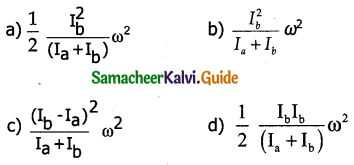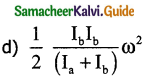Question 11.
The speed of the center of a wheel rolling on a horizontal surface is vo. A point on the rim in level with the center will be moving at a speed of speed of, _______.
a) 5:7
b) 2:3
c) 2:5
d) 7:5
d) 7:5

Question 12.
From a disc of radius R a mass M, a circular hole of diameter R, whose rim passes through the center is cut. What is the moment of inertia of the remaining part of the disc about a perpendicular axis passing through it _______. [NEET 2016]
a) 15MR²/32
b) 13MR²/32
c) 11MR²/32
d) 9MR²/32
b) 13MR²/32

Question 13.
The speed of a solid sphere after rolling down from rest without sliding on an inclined plane of vertical height h is, _______.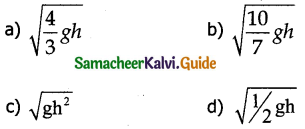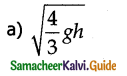Question 14.
The speed of the center of a wheel rolling on a horizontal surface is vo. A point on the rim in level with the center will be moving at a speed of speed of, _______. [PMT 1992, PMT 2003, IIT 2004]
a) zero
b) v0
c) $$\sqrt{2}$$v0
d) 2v0
c) $$\sqrt{2}$$v0

Question 15.
A round object of mass M and radius R rolls down without slipping along an inclined plane. The fractional force, _______.
a) dissipates kinetic energy as heat.
b) decreases the rotational motion.
c) decreases the rotational and transnational motion
d) converts transnational energy into rotational energy
d) converts transnational energy into rotational energyQuestion 1.
Define center of mass.
The center of mass of a body is defined as a point where the entire mass of the body appears to be concentrated.

Question 2.
Find out the center of mass for the given geometrical structures.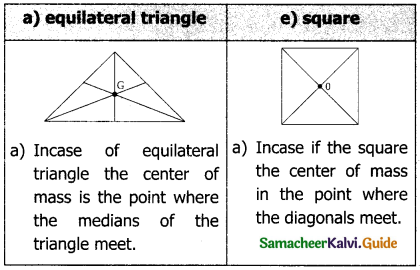b) Cylinder: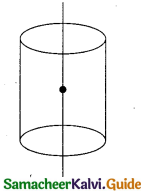The center of mass in case of the cylinder lies on the vertical axis and at the centre of the cylinder.

Question 3.
Define torque and mention its unit.
Torque is defined as the moment of the external applied force about a point or axis of rotation. The expression for torque is,
$$\vec{\tau}$$ = $$\vec{r}$$ x $$\vec{F}$$Question 4.
What are the conditions in which force cannot produce torque?
1) The torque is zero when r and P are either parallel or anti parallel
i.e θ = 0, if parallel sin θ = 0, τ = 0
θ = 180°, if anti parallel sin 180 = 0, τ = 0

2) The torque is zero if the force acts at the reference point as $$\vec { r }$$ = 0, τ = 0

Question 5.
Give any two examples of torque in day-to-day life.

• The opening and closing of a door about the hinges.
• Turning of a nut using a wrench.

Question 6.
What is the relation between torque and angular momentum?
Rate of change in angular momentum is equal to torque.
τ = $$\frac { d(L) }{ dt }$$

Question 7.
What is equilibrium?
A rigid body is said to be in mechanical equilibrium where both its linear momentum and angular momentum remain constant.

Question 8.
How do you distinguish between stable and unstable equilibrium?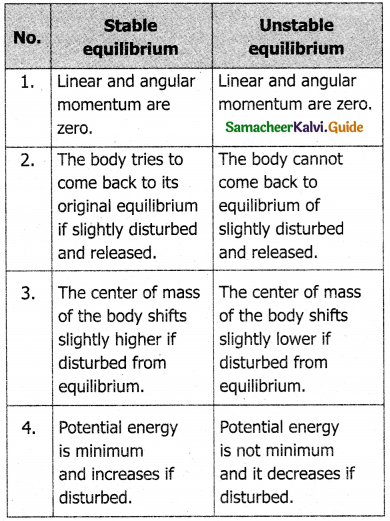Question 9.
Define couple.
A pair of forces which are equal in magnitude but opposite in direction and separated by a perpendicular distance so that their lines of action do not coincide that causes a turning effect is called a couple.

Question 10.
State principle of moments.
Principle of moments states that the sum of the clockwise moments is equal to sum of the anti-clockwise moments about the axis rotation.
d1f1 = d2f2Question 11.
Define center of gravity.
The center of gravity of a body is the point at which the entire weight of the body acts, irrespective of the position and orientation of the body.

Question 12.
Mention any two physical significance of the moment of Inertia.

• For rotational motion moment of Inertia is a measure of rotational Inertia.
• The Moment of Inertia of a body is not a variable quantity.

It depends not only on the mass of the body but also on the way the mass is distributed around the axis of rotation.

Question 13.
What is the radius of gyration?
The radius of gyration of an object is the perpendicular distance from the axis of rotation to an equivalent point mass, which would have the same mass as well as the same moment of inertia of the object.

Question 14.
State conservation of angular momentum?
When no external torque acts on a body, the net angular momentum of a rotating rigid body remains constant.

Question 15.
What are the rotational equivalents for the physical quantities?

1. mass
2. force

The rotational equivalents for (i) mass and (ii) force are moments of inertia and torque respectively.

Question 16.
What is the condition for pure rolling?
In the case of pure roiling, for all points on the edge, the magnitude of Vt Translation is V rotational are equal (Vtr = Vrot).
As V Trans = Vcm and Vrot = Rω, the condition
for pure rolling without slipping is Vcm = Rω.

Question 17.
What is the difference between sliding and slipping?
Sliding:

• Velocity of center of mass is greater than Rω i.e. VCM > Rω.
• Velocity of transnational motion is greater than velocity of rotational motion.
• Resultant velocity acts in the forward direction.

Slipping:

• Velocity of center of mass is lesser than Rω. i.e. VCM < Rω
• Velocity of translation motion is lesser than velocity of rotational motion.
• Resultant velocity acts in the backward direction.Question 1.
Explain the type of equilibrium with suitable examples.
The different types of equilibrium are:
i) Translational equilibrium – The body is set to be in translational equilibrium, when the linear momentum remains constant. The net force acting on body is zero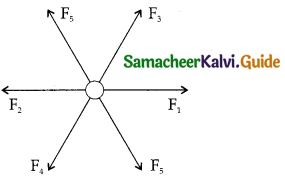Example: Let F1, F2, F3 …… Fn be n forces acting on body in different directions, and the net force on the body is the vector sum of different forces is the resultant, which is equal to zero, then the body is in translational equilibrium.

ii) Rotational equilibrium – The body is said to be in rotational equilibrium when the angular momentum is constant. Net Torque on the body is equal to zero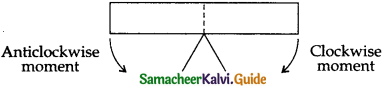Example: If a metre scale is balanced by a knife edge at its center then the body is in rotational equilibrium as clockwise moment is equal to Anticlockwise moment.

iii) Static equilibrium – When the linear momentum and angular momentum on body are zero , where no net force and no net torque, the body is in static equilibrium.
Example: A book kept in the table

iv) Dynamic equilibrium – Here linear momentum and angular momentum are kept constant. Net force and Net torque on a body is equal to zero.

v) Stable equilibrium:
Consider a china dish in which a small sphere is placed. It comes to rest at the bottom of the dish, said to be in stable equilibrium where potential energy is minimum. If displaced, the sphere tries to attain it original position after released. So the body is in stable equilibrium. In stable equilibrium.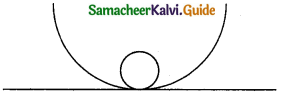a) Linear momentum and angular momentum are zero
b) The body tries to come back to equilibrium if slightly disturbed and released.
c) The centre of mass of the body shifts slightly higher if disturbed from equilibrium.
d) Potential energy is minimum and it is increased if disturbed.

Unstable equilibrium:
Consider an inverted china dish and place the sphere on the top of the dish. The body roll down to a new position if disturbed. It never returns to its original position stating unstable equilibrium. Incase of unstable equilibrium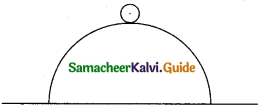a) Linear momentum and angular momentum are zero.
b) The body cannot come back to its original equilibrium if slightly disturbed and released.
c) The centre of mass of the body shifts slightly lower if disturbed from equilibrium.
d) potential energy is not minimum and it decreases if disturbed.

Neutral equilibrium:
Consider the sphere placed in a plane surface. The position of center of mass never changes, potential energy never changes if disturbed. So the equilibrium is said to be neutral equilibrium. In case of neutral equilibrium.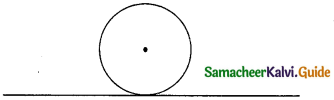a) Linear and angular momentum are zero.
b) The body remains at same equilibrium if slightly disturbed and released.
c) The center of mass of the body does not shift higher or lower if disturbed from equilibrium
d) Potential energy remains same even if disturbed.Question 2.
Explain the method to find the center of gravity of an irregularly shaped lamina.
The center of gravity of an irregularly shaped lamina by pivoting it at various points by trail and error. The lamina remains horizontal when pivoted at the point where the net gravitational force acts, which is the centre of gravity shown figure.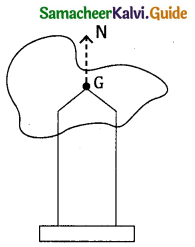When the body is supported at the centre for gravity, the sum of torques acting on all point masses of the rigid body becomes zero. Moreover the weight is compensated by the normal reaction force exerted by the pivot. The body in static equilibrium and hence it is horizontal.

Question 3.
Explain why a cyclist bends while negotiating a curve road. Arrive at the expression for angle of bending for a given velocity.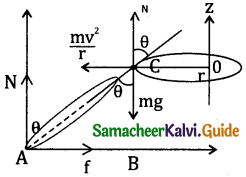Consider a cyclist negotiating a circular level road of radius ‘r’ with a velocity v. Cycle and cyclist are taken to be one system with mass m. The centre of gravity of the system is c and it goes in circle of radius r with centre o.
Consider OC as x – axis, vertical through 0 is z axis.
The system as a frame is rotating about z axis.
The system is at rest in the rotating frame.
centrifugal force on the system = $$\frac{\mathrm{mv}^{2}}{\mathrm{r}}$$
This force will act through centre of gravity.
The forces acting on the system are

1. Gravitational force (mg)
2. Normal force (N)
3. Frictional force (f)
4. Centrifugal force $$\frac{\mathrm{mv}^{2}}{\mathrm{r}}$$

As the system is in equilibrium in the rotational frame of reference, the net external force and external torque must be zero. Consider all torques about point A for rotational equilibrium.
τnet = 0.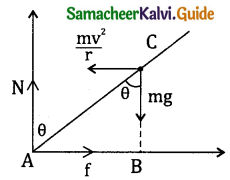Tongue due to gravitational force about point A = mg AB. Taken as negative as it produces clockwise momentum.
Torque due to centripetal force = $$\frac{\mathrm{mv}^{2}}{\mathrm{r}}$$(BC)
Taken as positive as it produces an anticlockwise moment.
-mg AB + $$\frac{\mathrm{mv}^{2}}{\mathrm{r}}$$ BC = 0
g AB = $$\frac{\mathrm{v}^{2}}{\mathrm{r}}$$ BC
from ∆ ABC
AB = AC sin θ
BC = AC cos θ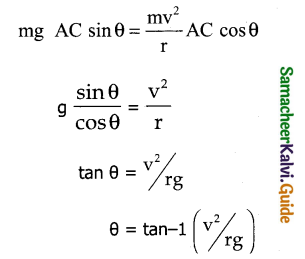Question 4.
Derive the expression for moment of inertia of a rod about its center and perpendicular to the rod.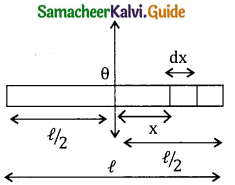Consider a uniform red of mass (m) and length $$(\ell)$$. Consider an axis passing through the geometric centre of the rod perpendicular to its length. Consider an infinitesimally small mass (dm) at a distance x from 0 origin of length dx. THe moment of Inertia (dl) of this mass (dm) about the axis is
dl = (dm)x²
As the mass is uniformly distributed, the mass per unit length (λ) if rod = λ = $$\frac{\mathrm{M}}{\ell}$$.
The mass of infinitesimally small length dx as
dm = λ dx = $$\frac{\mathrm{M}}{\ell}$$ dx
Moment of Inertria (I) of the entire rod can be obtained by integrating dl.
I = ∫ dI = ∫(dm) x² = ∫ $$\frac{\mathrm{M}}{\ell}$$x²dx
I = $$\frac{\mathrm{M}}{\ell}$$ ∫x² dx
As the mass is distributed on either side of the origin the limits for integration are taken from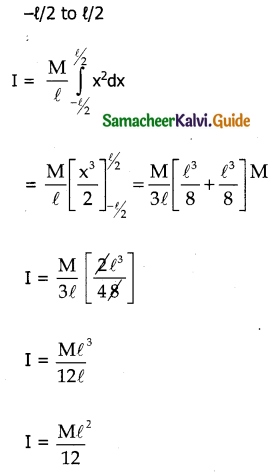Question 5.
Derive the expression for moment of Inertia of a uniform ring about an axis passing through the center and perpendicular to the plane.
Consider a uniform ring of mass ‘M’ and radius R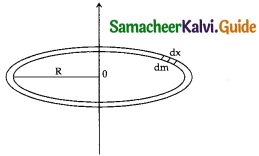Consider an axis passing through the center of the ring 0 and perpendicular to its plane take an infinitesimally small mass (dm) of length (dx) of the ring dm is located at a distance R from 0. The moment of Inertia (dl) of this small mass dm is
dI = (dm) R²
The length of the ring = Circumference of the ring $$(\ell)$$ = 2πR
As the mass is distributed uniformly
mass per unit length – λ = $$\frac {Mass}{length}$$ = $$\frac { MR }{ 2πR }$$
The mass of infinitesimally small length is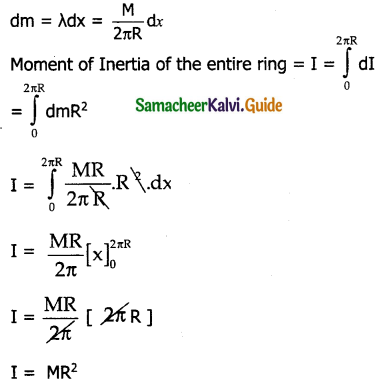Question 6.
Derive the expression for moment of inertia of a uniform disc about an axis passing through the center and perpendicular to the plane.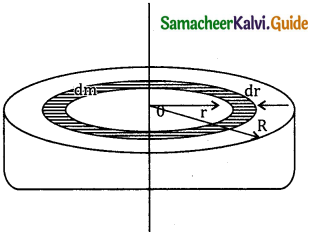Consider a disc of mass M and Radius R. This disc is made up of many infinitesimally small rings each of mass (dm) and thickness dr.
The moment of Inertia (dl) of this small ring is dl = (dm) r²
As the mass is uniformly distributed. The mass per unit area.
σ = $$\frac { Mass }{ Area }$$ = $$\frac{M R}{\pi R^{2}}$$
The mass of infinitesimally small ring is
dm = σ (2π r. dr) $$\frac{\mathrm{M}}{\pi \mathrm{R}^{2}}$$ 2 π r.dr
Where 2 π r.dr is the area of the elemental ring
∵ dm = $$\frac{2 \mathrm{M}}{\mathrm{R}^{2}}$$ rdr
∵ dI = $$\frac{2 \mathrm{M}}{\mathrm{R}^{2}}$$r³dr
∵ The moment of Inertia of the entire disc is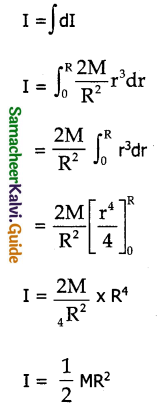Question 7.
Discuss the conservation of Angular momentum for example.
When no external torque acts on a body, the net angular momentum of a rotating rigid body remains constant. This is known as the law of conservation of angular momentum.
τ = $$\frac { dL }{ dt }$$
If τ = 0
then L is a constant.
As angular momentum L = Iω, the conservation of angular momentum can be written as
Iiωi = Ifωf
Iω = constant
The above equation indicates if I increase ω will decrease and vice versa, to keep angular momentum constant.

Example:
Let us consider an ice dancer. The dancer spins slowly when the hands are stretched out and spins faster when the hands are brought close to the body.

Stretching the hands away from body increases the moment of Inertia, thus angular velocity decreases resulting in a slower spin. When the hands are brought to the body the moment of Inertia decreases and thus angular velocity in-creases resulting in faster spin.Question 8.
State and prove parallel axes theorem.
Law:
Parallel axes theorem states that the moment of Inertia of a body about any axis is equal to the sum of its moment of Inertia about a parallel axis through its center of mass and the product of the mass of the body and the square of the perpendicular distance between two axis.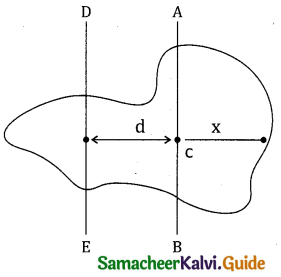Let Ic be the moment of Inertia of the body of mass m about an axis (AB) passing through the center of mass.
Let I be the moment of Inertia of a parallel axis (DE) at a distance d from AB is
I = Ic + Md²
Proof:
Consider a rigid body as in figure. Let Ic be the moment of Inertia of the body about an axis AB passing through center of mass c.
DE is another axis parallel to AB at a perpendicular distance d from AB. Let I be the moment of Inertia about the axis DE.
Consider a point P of mass ‘m’ on the body at a distance, x from c.
The moment of Inertia of the point mass about the axis DE = m(x+d)².
The moment of Inertia I of the whole body about
DE = I = ∑ m (x+d)²
I = ∑ m (x² + d² + 2xd)
I = ∑ mx² + md² + 2mxd
I = ∑ mx² + ∑ md² + 2d ∑ mx
Here ∑ mx² = moment of Inertia of the body about the center of mass
∴ Ic = ∑ mx²
The term ∑mx = 0, because x can take positive and negative values w.r. to the axis AB.
I = Ic + Imd²
but ∑m = M
∵ I = Ic + Md²
Hence proved

Question 9.
State and prove perpendicular axis theorem.
Statement:
Perpendicular axes theorem states that moment of Inertia of a plane laminar body about an axis perpendicular to its plane is equal to the sum of moments of Inertia about two perpendicular axes lying in the plane of the body such that all the three axes are mutually perpendicular and have a common point.
Let x, y axes lie in the plane and Z-axis perpendicular to one plane of the laminar object. If the moment of inertia of the body about x and y axes are lx and Iy respectively and Iz is the Moment of Inertia about the z-axis, then the perpendicular axes theorem can be expressed as Iz = Ix + Iy
Proof: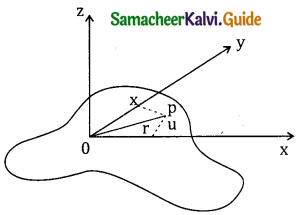Consider a plane laminar objects of negligible thickness on which lies the origin o. X and Y axes lie on the plane and Z-axis is perpendicular to the plane. The lamina is considered to be made up of large number of particles of mass m. Let us consider one such particle at a point P which has co-ordinates (x,y) at distance r from o.
The moment of Inertia of the particle about the z-axis is Iz = mr².
The summation of the above equation gives the Moment of Inertia of the entire lamina about z-axis = Iz = I mr².
Here r² = x² + y²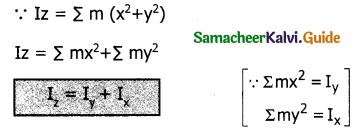Hence proved.Question 10.
Discuss rolling on an inclined plane and arrive at the expression for the acceleration.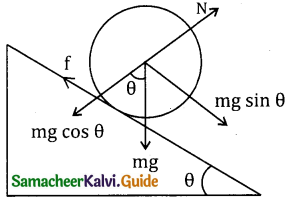Consider a round object of mass m and radius R is rolling down an inclined plane without slipping.
There are two forces acting on the object along the inclined plane.
One is the component of gravitational force mg sin θ and the other is the static frictional force ‘f’.
The other component of gravitational force mg cos θ is cancelled by the normal force N exerted by the plane.
Considering free body diagram for the inclined plane.
For translational motion, mg sin 0 is the supporting force and f is the opposing force
mg sin θ – f = ma → (1)
For rotational motion, we have to consider the torque w.r. to the centre of the object. Here mg sin 0 cannot cause torque is it passes through it, so frictional force causes the torque.
τ = I∝ = fR → (2)
W.K.T a =R∝ and moment of Inertia I = mk²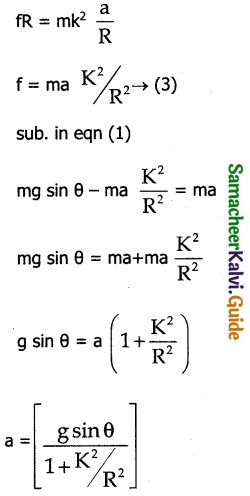IV. Conceptual Questions:

Question 1.
When a tree is cut, the cut is made on the side facing the direction in which the tree is required to fall why?
The weight of the tree exerts a torque about the point where the cut is made. This causes the rotation of the tree about the cut. So the reason why the cut is made on the side facing the direction of fall.

Question 2.
Why does a porter bend forward while carrying a sack of rice on his back?
When porter is carrying a sack of rice, on his back, the position of center of gravity changes. In order to bring it in the middle for stable equilibrium, porter bends forward.

Question 3.
Why is it much easier to balance a meter scale on your fingertip than balancing on a match stick?
It is much easier to balance a meter scale on your finger than balancing on a match stick. While balancing the meter scale in stable equilibrium. The vertical line passes through the center of gravity of scale should pass through the base of supporting material. The finger has more base over than a match stick.Question 4.
Two identical water bottles one empty and the other filled with water are allowed to roll down an inclined plane. Which one of them reaches the bottom first? Explain.
Empty water bottle has large moment of Inertia than filled water bottle. So least value of radius of gyration is for bottle filled with water. So the water bottle filled with water reaches bottom first.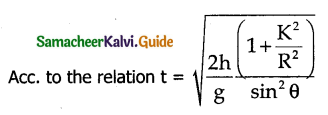Suggests that for a given angle of inclination, the object with least value of radius of gyration k reach the bottom of incline first.

Question 5.
Write the relation between angular momentum and rotational K.E. Draw a graph for the same. For two objects of same angular momentum compare the moment of Inertia using the graph.
Angular momentum = L = Iω
Rotational K.E = KR = $$\frac { 1 }{ 2 }$$Iω²
By comparing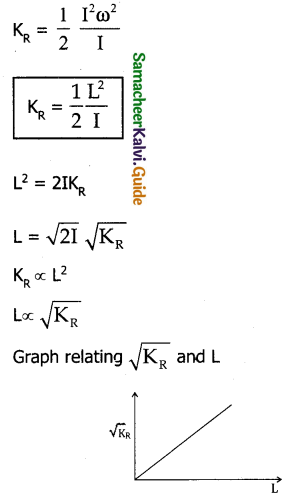The shape of the graph is a straight line. The $$\frac { 1 }{ slope }$$ of the curve gives the value of the moment of Inertia I. If angular momentum of two objects are same, rotational K.E one same having same moment of Inertia.

Question 6.
A rectangle block rests on a horizontal table. A horizontal force is applied on the block at a height h above the table to move the block. Does the line of action of the normal force N exerted by the table on the block depend on h?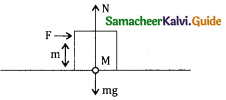Yes, the normal force is concentrated at the center of mass. When force F is applied normal reaction of the floor shifts to right the block topples when N reaches edge.

Question 7.
Three identical solid spheres move down through three inclined planes A, B, and C all same dimensions. A is without friction, B is undergoing pure rolling and C is rolling with slipping compare the kinetic energies EA, EB and Ec at the bottom.
A possesses translational K.E
B possesses sum of rotational K.E + Translational K.E
C possesses more rotation than translational KE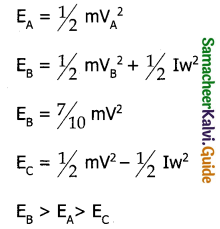Question 8.
Give an example to show that the following statement is false. “Any two forces acting on a body can be combined into single force that would have same effect”
Any two forces acting an a body can be combined to form a single force then it is called a resultant. In some cases effect of resultant is not same as the effect of the two forces acting

Example: A large body can be considered as made of a number of mass particles and all mass particles interact with each other. But the vector sum of all these internal forces is zero.V. Numerical Problems:

Question 1.
A uniform disc of mass 100g has a diameter of 10cm. Calculate the total energy of the disc when rolling along a horizontal table with a velocity of 20 cms-1. (take the surface of the table as reference)
Solution:
m = 100 x 10-3 kg = 100g
2r = 10 x 10-2 m r = 5 cm
r = 5 x 10-2 m v = 20 cm/s
V = 20 cms-1= 20 x 10-2 m/s
E = ?
for rolling body total K.E is
= $$\frac { 1 }{ 2 }$$ mv² + $$\frac { 1 }{ 2 }$$ Iw²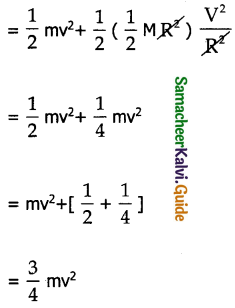K.E = 3/4 x 100 x 20 x 20 x 10-3 x 10-4
= $$\frac { 3 }{ 4 }$$ x 10-3 x 4
= 3 x 10-3 J

Question 2.
A particle of mass 5 units in moving with a uniform speed of v = 3$$\sqrt{2}$$ units in the xoy plane alone the line y = x+4. Find the magnitude of angular momentum
Solution:
y = x + 4
Comparing it with straight line equation y = mx + c
m = tan θ = 1 θ = 45°
A line makes an angle θ = 45° with five x axis and intercepts on the y axis is + 4.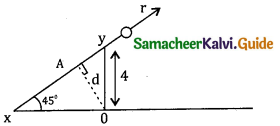Draw a ⊥ r from 0 to the line y = x + 4
OA = d = 4sing 45 = $$\frac{4}{\sqrt{2}}$$
Angular momentum about 0 = Linear momentum x ⊥ r distance from 0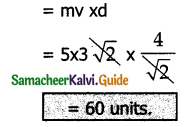Question 3.
A fly wheel rotates with a uniform angular acceleration. If its angular velocity increases form 20 π rad /s to 40 π rad/s in 10 seconds. Find the number of rotations in that period.
Solution:
w = θ/t
θ = wt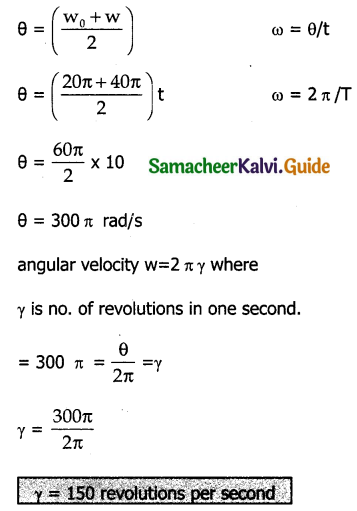Question 4.
A uniform rod of mass m and length l makes a constant angle θ with an axis of rotation which passes through one end of the rod. Find the moment of Inertia about this axis.
Solution: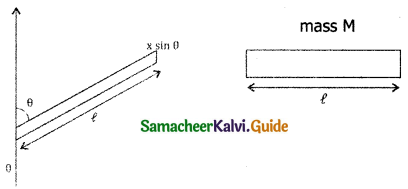Here mass is distributed on length taking an element of small length dx at a distance x as in figure.
dm = $$\frac{\mathrm{M}}{\ell}$$.dx
Moment of Inertia of small element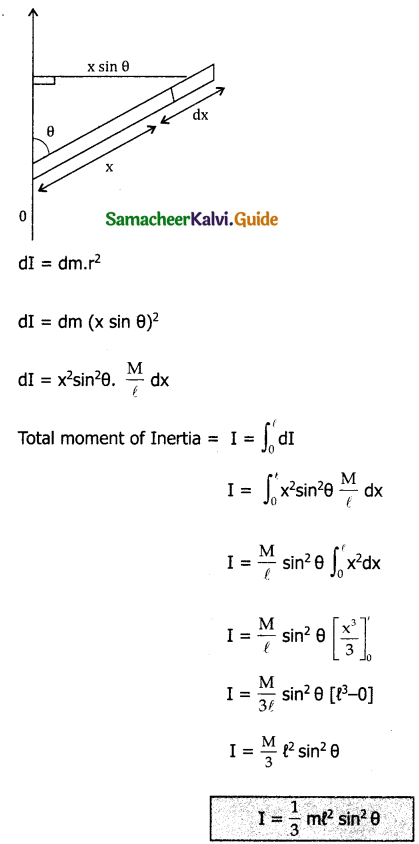Question 5.
Two particles P and Q of mass 1 kg and 3 kg respectively start moving towards each other from rest under mutual attraction. What is the velocity of their center of mass?
Solution: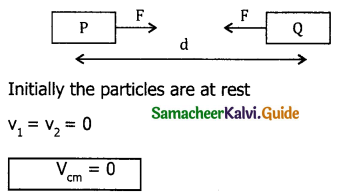Since external force acting as them is zero, the velocity of cm is constant and hence Vcm =0, whenever the separation.

Question 6.
Find the moment of Inertia of a hydrogen molecule about an axis passing through its center of mass and perpendicular to the inter atomic axis. Given mass of H2 atom = 1.7 x 10-27 kg and inter atomic distance is equal to 4 x 10-10 m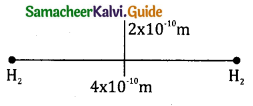Solution :
Mass of each H2 atom = 1.7 x 10-27 kg
Distance of each H2 atom from the axis of rotation = 2 x 10-10 m
Moment of Inertia about the axis = I = mr² + mr²
I = 2mr²
I = 2 x 1.7 x 10-27 x (2 x 10-10)mr²
= 13.6 x 10-47 kgm²
I = 13.6 x 10-47 kgm²
I = 1.36 x 10-46 kgm²Question 7.
On the edge of a wall, we build a brick tower that only holds because of the bricks own weight. Our goal is to build a stable tower whose overhang d is greater than the length l of a single brick. What is the minimum number of bricks you need?
(Hint: find the center of mass for each brick and add)
Solution: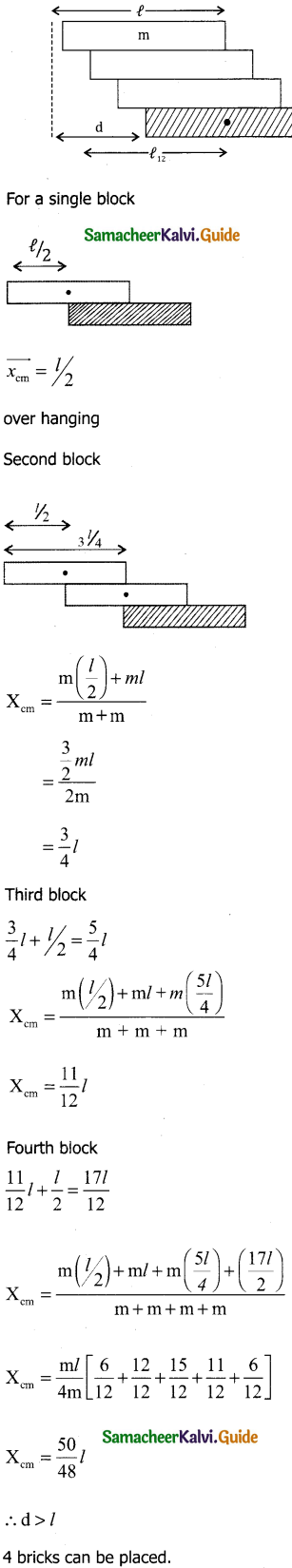Question 8.
The 747 being plane is landing at a speed of 70 m/s. Before touching the ground the wheels are not rotating. How long a skid mark do the wing wheel leave (assume their mass is 100 kg which is distributed uniformly radius is 0.7m. Weight supported by back wheel is 232KN and coefficient of friction with the ground is 0.5?)
Solution: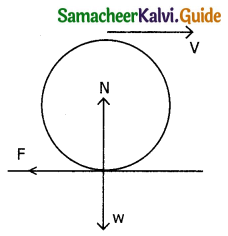Force acting on the wheel after the plane touches the surface.
(Note: 747 has 16 wheels below wings and 2 wheels below nose)
If the wheel is not accelerating
N = W
τ = fR
But f = µN = µW
τ = µWR
Angular acceleration x = $$\frac { τ }{ I }$$ = $$\frac{\mu \mathrm{WR}}{\frac{1}{2} \mathrm{MR}^{2}}$$
∵ τ = Iα
= $$\frac { 2 µ W }{ MR }$$
The time taken by the bowing wheel to stop skidding.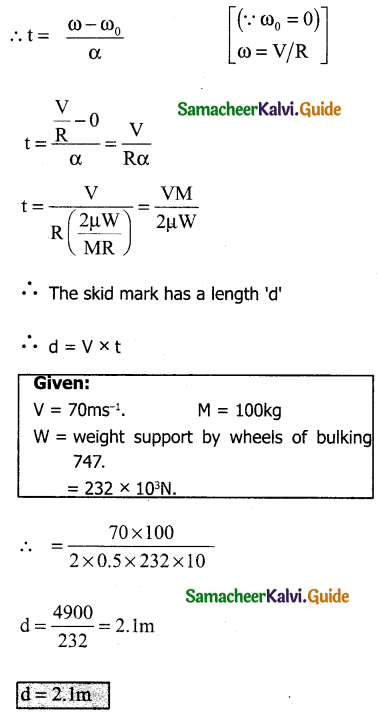Part – II:

### 11th Physics Guide Motion of System of Particles and Rigid Bodies Additional Important Questions and Answers

I. Multiple choice questions:

Question 1.
The changes produced by the deforming forces in a rigid body are
(a) very large
(b) infinity
(c) negligibly small
(d) small
(c) negligibly small

Question 2.
Particles of masses m, 2m, 3m …………. nm are placed on the same line at distances L, 2L, 3L ………… nL from 0. The distance of centre of mass from 0 is _______.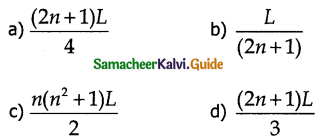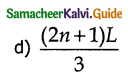Question 3.
For bodies of regular shape and uniform mass distribution, the center of mass is at –
(a) the comers
(b) inside the objects
(c) the point where the diagonals meet
(d) the geometric center
(d) the geometric centerQuestion 4.
Look at the drawing given in the figure which has been drawn with ink of uniform line thickeners. The mass of ink used to draw each of the two inner circles and each of the two line segments is m. The mass of ink used to draw outer circle is 6m. The coordinates of the centres of different parts are outer circle (0, 0), left inner circle (-a, a) right inner circle (a, a) vertical line (0, 0) and horizontal line (o, -a). The ‘y’ co-ordinate of the centre of mass ink in this drawing is _______.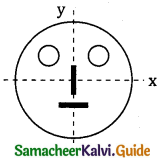a) $$\frac { a }{ 10 }$$
b) $$\frac { a }{ 8 }$$
c) $$\frac { a }{ 12 }$$
d) $$\frac { a }{ 3 }$$
a) $$\frac { a }{ 10 }$$

Question 5.
Center of mass may lie-
(a) within the body
(b) outside the body
(c) both (a) and (b)
(d) only at the center
(c) both (a) and (b)

Question 6.
The mass per unit length of a non uniform rod of length L is given by μ = λx² Where A. is a constant and λ is the distance from one end of the rod. The distance of mass of rod from this end is _______.
a) L/2
b) L/4
c) $$\frac { 3L }{ 4 }$$
d) L/3
c) $$\frac { 3L }{ 4 }$$

Question 7.
The motion of center of mass of a system of two particles is unaffected by their internal forces-
(a) irrespective of the actual directions of the internal forces
(b) only if they are along the line joining the particles
(c) only if acts perpendicular to each other
(d) only if the acting opposite
(a) irrespective of the actual directions of the internal forcesQuestion 8.
A cubical block of ice of mass m and edge L is placed on a tray of mass M. If the ice melts, by what distance the center of mass (ice + tray) system descend?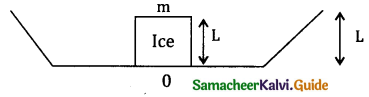a) $$\frac { mL }{ m+m }$$
b) $$\frac { mL }{ 2(m+m) }$$
c) $$\frac { mL }{ m }$$
d) $$\frac { ML }{ m }$$
b) $$\frac { mL }{ 2(m+m) }$$

Question 9.
The center of mass of a system of particles does not depend on
(a) masses of particles
(b) position of the particles
(c) distribution of masses
(d) forces acting on the particles
(d) forces acting on the particles

Question 10.
Two bodies with K.E in the ratio 4:1 are moving with equal linear momentum. The ratio of their masses is _______.
a) 1:2
b) 1:1
c) 1:1
d) 1:4
b) 1:1

Question 11.
Three particles A, B and C of masses m, 2m and 3m are placed on a straight line of length 2L as shown. The moment of inertia about an axis passing through B and perpendicular to line AC is _______.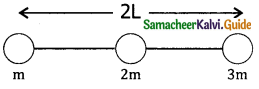a) ML²
b) 2ML²
c) 3ML²
d) 4ML²
d) 4ML²

Question 12.
Three thin, uniform, identical rods each of mass M and length L are Joined as shown. The moment of inertia about an axis passing through 0 and perpendicular to plane is _______.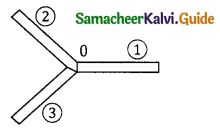a) 3ML²
b) ML²
c) $$\frac{M L^{2}}{3}$$
d) zero
b) ML²

Question 13.
The distance between the center of carbon and oxygen atoms in the gas molecule is 1.13 A. The center of mass of the molecule relative to the oxygen atom is-
(a) 0.602 Å
(b) 0.527 Å
(c) 1.13 Å
(d) 0.565 Å
(b) 0.527 Å
Given,
Inter atomic distance = 1.13 Å
Mass of carbon atom = 14
Mass of oxygen atom = 16
Let C.M. of molecule lies at a distance of X from oxygen atom-
i.e. m1r1 = m2r2
16 X = 14(1.13 – X)
30 X = 15.82
X = 0.527 Å

Question 14.
From a thin uniform disc of radius 2R, another disc of diameter 2R is removed. The mass of the remaining portion is m. Find the moment of Inertia of the shaded portion about an axis passing through 0 and perpendicular to the plane.
a) $$\frac{8 M R^{2}}{13}$$
b) $$\frac{ M R^{2}}{6}$$
c) $$\frac{13 M R^{2}}{6}$$
d) $$\frac{8 M R^{2}}{6}$$
c) $$\frac{13 M R^{2}}{6}$$

Question 15.
The sum of moments of masses of all the particles in a system about the center of mass is-
(a) minimum
(b) maximum
(c) zero
(d) infinity
(c) zeroQuestion 16.
The radius of gyration of a system of particle about an axis passing through the center of mass and perpendicular to the line joining the particles. The system consists of two particle of masses m1 and m2 placed at separation r is _______.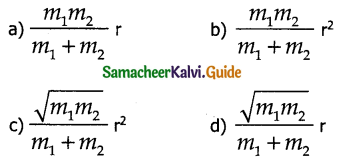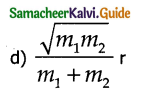Question 17.
A fly wheel rotates with a uniform angular acceleration. Its angular displacement increases from 2 n radians to 10 n radians in 4s. The number of revolutions is this period _______.
a) 10
b) 12
c) 6
d) 5
b) 12

Question 18.
The reduced mass of the system of two particles of masses 2 m and 4 m will be –
(a) 2 m
(b) $$\frac {2 }{ 3 }$$y m
(c) $$\frac {3}{ 2 }$$y m
(d) $$\frac { 4 }{ 3 }$$m
(d) $$\frac { 4 }{ 3 }$$m

Question 19.
A pulley has the shape of a uniform solid disc of mass 2kg and radius 0.5m. A string is wrapped over its rim and is pulled by force of 2.5N. The pulley is free to rotate about its axis. Initially the pulley is at rest. Find the angular velocity and angle rotated by pulley in 10S _______.

Question 20.
A solid sphere of radius R lies on a smooth horizontal surface. It is pulled by a horizontal force acting tangentially from the highest point. Find the distance travelled by the sphere during the time it make one rotation?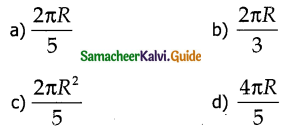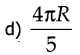Question 21.
From a circular disc of mass M and radius R a part of 60° is removed. The M.I of the remaining portion of disc about an axis passing through the center and perpendicular to the plane of disc is _______.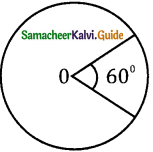a) 5/6 MR²
b) 5/12 MR²
c) 5/3 MR²
d) 5/24 MR²
b) 5/12 MR²

Question 22.
If I0 is the Moment of Inertia of a body about an axis passing through its centre of mass. The M.I. of the parallel axis and at a distance d is I = I0 + Md². The variation of I with d is _______.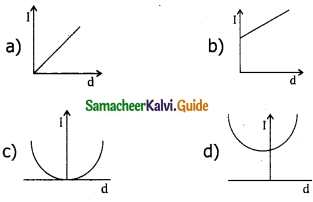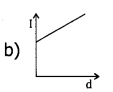Question 23.
A rod of length L and mass M0 is bent to form a semi-circular ring as shown. The M. I. about XX1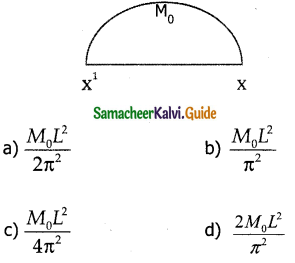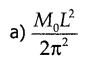Question 24.
Infinitesimal quantity means –
(a) collective particles
(b) extremely small
(c) nothing
(d) extremely larger
(b) extremely smallQuestion 25.
A particle performs uniform circulation motion with an angular momentum L. If the frequency of the particle motion is doubled and its kinetic energy is halved the angular momentum becomes _______.
a) 2L
b) 4L
c) L/2
d) L/4
d) L/4

Question 26.
A thin horizontal circular disc is rotating about a vertical axis passing through its center. An insect is at rest at the point near the rim of the disc. The insect now moves along a diameter of the disc to reach other end. During the journey of the insect the angular speed of the disc.
a) remains unchanged
b) continuously decreases
c) continuously increases
d) first increases and then decreases
d) first increases and then decreases

Question 27.
A solid disc rolls clockwise without slipping over a horizontal path with a constant speed V. Then the magnitude of velocities of points A, B & C (in figure) with respect to a standing observer are respectively _______.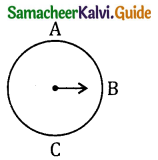a) V, V and V
b) 2V, $$\sqrt{2}$$, zero
c) 2V, 2V, zero
d) 2V, $$\sqrt{2}$$, $$\sqrt{2}$$ V
b) 2V, $$\sqrt{2}$$, zero

Question 28.
Torque is given as –
(a) $$\vec{r}$$ . $$\vec{F}$$
(b) $$\vec{r}$$ x $$\vec{F}$$
(c) $$\vec{F}$$ x $$\vec{r}$$
(d) r F cos θ
(b) $$\vec{r}$$ x $$\vec{F}$$

Question 29.
A solid sphere of mass m and radius R rolls without slipping an the horizontal surface such that Vcm = V0
a) The K.E of rotation is 1/5 mV0
b) The total K.E in 7/10 mV0²
c) The mechanical energy is mgh + 7/10 mV0²
d) All are correct
d) All are correct

Question 30.
The direction of torque ácts –
(a) along $$\vec{F}$$
(b) along $$\vec{r}$$ & $$\vec{F}$$
(c) Perpendicular to $$\vec{r}$$
(d) Perpendicular to both $$\vec{r}$$ & $$\vec{F}$$
(d) Perpendicular to both $$\vec{r}$$ & $$\vec{F}$$Question 1.
Explain center of mass for distributed point masses.
A point mass is a hypothetical point particle has non zero mass and no size or shape.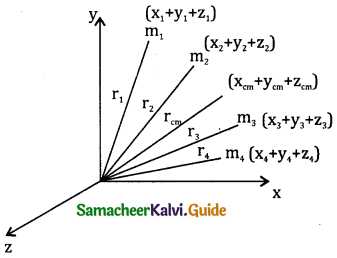Let m1, m2, m3 …. mn be the point masses with coordinates as x1, x2, x3 ………. x4 as co-ordinates of
particle of these point masses in the x-direction from origin. The equation for x coordinate of the center of mass is
Xcm = $$\frac{\sum m_{i} x_{i}}{\sum m_{i}}$$
Xmi is the total mass ‘M’ of all particles ∑mi = m.
Hence
Xcm = $$\frac{\sum \mathrm{m}_{\mathrm{i}} \mathrm{x}_{\mathrm{i}}}{\mathrm{M}}$$
for y and z coordinates of the center of mass for these distributed point masses
Ycm = $$\frac{\sum \mathrm{m}_{\mathrm{i}} \mathrm{y}_{\mathrm{i}}}{\mathrm{M}}$$
Zcm = $$\frac{\sum \mathrm{m}_{\mathrm{i}} \mathrm{z}_{\mathrm{i}}}{\mathrm{M}}$$
Hence the position of center of mass of these point masses in Cartesian coordinate system is (xcm + ycm + zcm )
In general the position of center of mass can be written in vector from as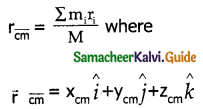is the position vector of center of mass and ri = xi$$\hat{i}$$ + yi$$\hat{j}$$+zi$$\hat{k}$$ is the position vector of distributed masses where i, j, k are unit vectors along x, y and z axis respectively.

Question 2.
Explain center of mass of a two-particle system under different cases. Let us consider two-particle system having masses m1 and m2 at positions x1 and x2 respectively along x-axis. The center of mass can be determined in the following 3 ways based on the choice of the coordinate system.
1) When the masses are an the positive x-axis.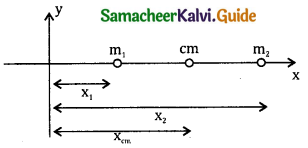The origin in taken arbitrarily so the masses m1 and m2 are at positions x1 and x2 on positive x-axis. Then the center of mass will also be in the positive x-axis at x cm given by
Xcm = $$\frac{\mathrm{m}_{1} \mathrm{x}_{1}+\mathrm{m}_{2} \mathrm{x}_{2}}{\mathrm{~m}_{1}+\mathrm{m}_{2}}$$

2) When the origins coincides with any one of the masses:
When the origin coincides with the point mass m1 its position x1 = 0 then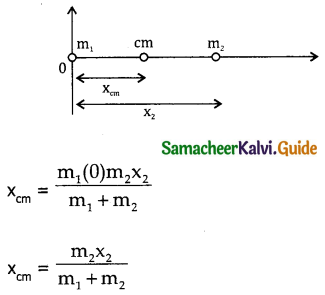3) When the origin coincides with the center of mass it self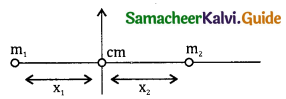If the origin is made to coincide with center of mass then xcm = 0.
The mass m1 is on the negative x-axis
Hence position of x1 is negative.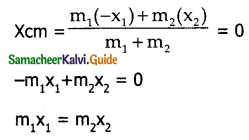This equation is known as principle of moments.

Question 3.
Show that in the absence of any external force the velocity of center of mass remains constant.
Suppose an external force Fext acts an a system of mass M and produces an acceleration. acm in its centre of mass. Then
$$\vec { \tau }$$ext = Ma$$\overline { cm }$$
in the absence of external forces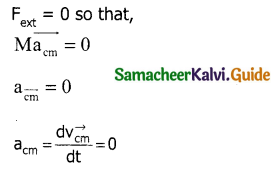∴ $$\vec { V }$$cm = constant. Where $$\vec { V }$$cm is the velocity at the center of mass

Question 4.
Write expressions for velocity and acceleration at the center of mass.
Let $$\vec { V }$$cm and a$$\overline { cm }$$ be the velocity and acceleration at the center of mass respectively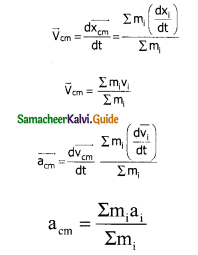Question 5.
Obtain the relation between torque and angular acceleration.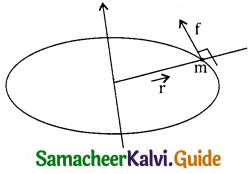Consider a rigid body rotating about a fixed axis. A point mass’m’ in the body will execute circular motion about the fixed axis. Let ‘f’ be the force acting on the point mass, produces necessary, torque for this rotation. This force $$\vec { f }$$ is perpendicular to $$\vec { r }$$.
The torque produced by the force on the point mass m about the axis
τ = $$\vec { r }$$ x $$\vec { f }$$ = rf sin 90
τ = rf
τ = r.ma
= r m (r∝)
= mr²∝
But mr² = I = moment of Inertia
Since a rigid body is made up of many such point masses, the moment of Inertia is the sum of moment of Inertia of all such individual point masses
I = ∑miri² i
τ = ( ∑miri²).∝
τ = I∝Question 6.
Explain principle of moments and state mechanical advantage.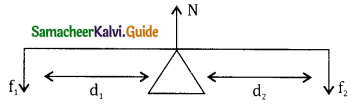Consider a light rod of invisible mass which is pivoted at a point along its length. Let two parallel force f1 and f2 act at the two ends at distances, d1 and d2 from the point of pivot and normal reaction force N at the point of Pivot. In order to make the red stationary in horizontal position in should be in translational and rotational equilibrium ie both net force and net torque must be zero.
For Net force zero
N-f1f2 = 0
N = f1 + f2 → (1)
For net torque to be zero
f1d1 – f2d2 = 0
f1d1 = f2d2 → (2)
The equation represents principle of moments, ie sum of clockwise moments. Should be equal to sum of anticlockwise moments
Equation (2) can be written as
$$\frac{f_{1}}{f_{2}}$$ = $$\frac{d_{2}}{d_{1}}$$
Here the radio $$\frac{d_{2}}{d_{1}}$$ = Mechanical advantage.
F1 is called load and F2 taken as effort. M.A should be always greater than 1 in F1 > F2 so that for less amount of effort, large load can be lifted.

Question 7.
What is a rigid body?
A rigid body is one which maintains its definite and fixed shape even when an external force acts on it.

Question 8.
Why do we prefer to use a wrench of longer arm?
The torque applied as the nut by the wrench is equal to the force multiplied by the perpendicular distance from the axis of rotation. Hence to increase torque a wrench of longer arm is preferred.

Question 9.
A cat is able to land on its feet after a fall. Which principle of physics is being used? Explain.
A cat is able to land on its feet after a fall. This is based on the law of conservation of angular momentum. When the cat is about to fall, it curls its body to decrease the moment of inertia and increase its angular velocity. When it lands it stretches out its limbs. By which it increases its moment of inertia and inturn decreases its angular velocity. Hence, the cat lands safely.

Question 10.
The bottom of the ship is made heavy. Why?
The bottom of the ship is made heavy so that the centre of gravity remains low. This ensures stability.Question 11.
What is the advantage of fly wheel?
In case of a fly wheel the whole mass is practically near the nm which is situated for away from the axis of rotation. This is done to increase the moment of inertia of the wheel there by making the motion smooth and less Jerkey.

Question 12.
There is a stick half of which is wooden and half is of steel. It is pivoted at the wooden end and a force is applied at the steel end at right angles to the length. Next it is pivoted at steel end and same force is applied at the wooden end. In which case it the angular acceleration more and why?
The distribution of mass faster away in first case compared with second case. So moment of Inertia is more in first case than second case. Applied torque is same in both cases. As τ =I∝, ∝ = τ/I, and ∝ = 1/I. So angular acceleration is less in first case than second.

Question 13.
Can the couple act on a rigid body produce translator motion?
No. It can produce only rotatory motion.

Question 14.
A particle performing uniform circular motion has angular momentum L. what will be new angular momentum if its angular frequency is doubled.
Kinetic energy halved?
Rot K.E = k = 1/2 Iw²
∵ I = $$\frac{2 k}{w^{2}}$$
Angular momentum
L = Iw = $$\frac{2 k}{w^{2}}$$ w = $$\frac { 2k }{ w }$$
when the angular frequency in doubled and K.E is halved the angular momentum becomes.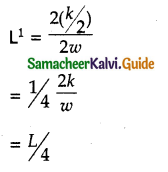Question 15.
How will you distinguish between a hand boiled egg and a raw egg by spinning it on a table top?
Hand boiled egg acts just like a rigid body while rotating while not in the case of raw egg to the liquid matter present in it. In raw egg the liquid water tries to go away from the center, there by increasing moment of Inertia. As the moment of inertia increases the raw egg will spin with less angular acceleration provided same torque applied in both cases thus hand boiled egg will spin faster.Question 16.
From a uniform disc of radius R, a circular disc of radius R / 2 is cut out. The center of the hole is at R / 2 from the center of original disc. Locate the center of gravity of the resultant flat body.
∵ v = $$\sqrt{\frac{2 E}{m}}$$